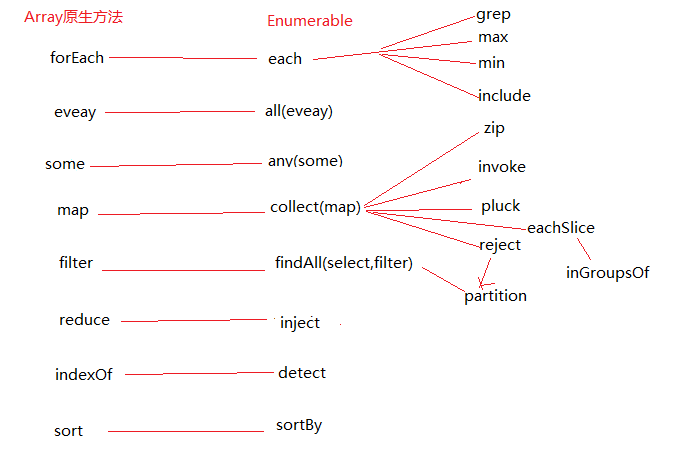# 【Javascript】Prototype源码浅析—Enumerable部分(二)

2012-01-17

``````    var array_1 = [1,2,3];
var array_2 = ['a','b','c'];
(function(){
for(var i = 0, len = array_1.length; i < len; i++){
for(var j = 0, len_j = array_1.length; i < len_j; j++){
if('c' === array_2[j]){
break;
}
console.log(array_2[j]);
}
}
})();//a,b,a,b,a,b
(function(){
for(var i = 0, len = array_1.length; i < len; i++){
try{
for(var j = 0, len_j = array_1.length; i < len_j; j++){
if('c' === array_2[j]){
throw new Error();
}
console.log(array_2[j]);
}
}catch(e){
console.log('退出一层循环');
}
}
})();//a,b,'退出一层循环',a,b,'退出一层循环',a,b,'退出一层循环'
(function(){
try{
for(var i = 0, len = array_1.length; i < len; i++){
for(var j = 0, len_j = array_1.length; i < len_j; j++){
if('c' === array_2[j]){
throw new Error();
}
console.log(array_2[j]);
}
}
}catch(e){
console.log('退出一层循环');
}
})();//a,b,'退出一层循环'
``````

``````    Enumerable.each = function(iterator, context) {
var index = 0;
try{
this._each(function(value){
iterator.call(context, value, index++);
});
}catch(e){
if(e != \$break){
throw e;
}
}
return this;
};
``````

Enumerable中的某些方法在一些现代浏览器里面已经实现了（参见chrome原生方法之数组），下面是一张对比图：检测：all any include

all : 若 Enumerable 中的元素全部等价于 true，则返回 true，否则返回 false

``````  function all(iterator, context) {
var result = true;
this.each(function(value, index) {
result = result && !!iterator.call(context, value, index);
});
return result;
}
``````

对于all方法来说，里面的两个参数都不是必须的，所以，内部提供了一个函数，以代替没有实参时的iterator，直接返回原值，名字叫做Prototype.K。Prototype.K的定义在库的开头，是一个返回参数值的函数Prototype.K = function(x){return x;}。另外，在all方法中，只要有一个项的处理结果为false，整个过程就可以放弃（break）了，于是用到了本文开头的中断循环的方法。最后的形式就是：

``````  Prototype.K = function(){};
Enumerable.all = function(iterator, context) {
iterator = iterator || Prototype.K;
var result = true;
this.each(function(value, index) {
result = result && !!iterator.call(context, value, index);
if (!result) throw \$break;
});
return result;
}
``````

``````  function collect(iterator, context) {
iterator = iterator || Prototype.K;
var results = [];
this.each(function(value, index) {
results.push(iterator.call(context, value, index));
});
return results;
}
``````

any：若 Enumerable 中的元素有一个或多个等价于 true，则返回 true，否则返回 false，其原理和all差不多，all是发现false就收工，any是发现true就收工。

``````  function any(iterator, context) {
iterator = iterator || Prototype.K;
var result = false;
this.each(function(value, index) {
if (result = !!iterator.call(context, value, index))
throw \$break;
});
return result;
}
``````

include：判断 Enumerable 中是否存在指定的对象，基于 == 操作符进行比较

``````  function include(object) {
if (Object.isFunction(this.indexOf))//这个判定函数应该很熟悉了
if (this.indexOf(object) != -1) return true;//有indexOf就直接调用

var found = false;
this.each(function(value) {//这里的效率问题
if (value == object) {
found = true;
throw \$break;
}
});
return found;
}
``````

 返回单个元素：max min detect
 返回一个数组：grep findAll reject partition

max(iterator, context)依旧可以带有两个参数，可以先用iterator处理之后再来比较值，这样的好处就是不必局限于特定的数据类型，比如，对象数组按照一定规则取最大值：

``````    console.dir([{value : 3},{value : 1},{value : 2}].max(function(item){
return item.value;
}));//3
``````

``````  function max() {
var result;
this.each(function(value) {
if (result == null || value >= result) //result==null是第一次比较
result = value;
});
return result;
}
``````

``````  function max(iterator, context) {
iterator = iterator || Prototype.K;
var result;
this.each(function(value, index) {
value = iterator.call(context, value, index);
if (result == null || value >= result)
result = value;
});
return result;
}
``````

min的原理也一样。

detect和any的原理和接近，any是找到一个true就返回true，detect是找到一个true就返回满足true条件的那个值。源码就不贴了。

grep 这个很眼熟啊，一个unix/linux工具，其作用也很眼熟——就是返回所有和指定的正则表达式匹配的元素。

``````    Enumerable.grep = function(filter) {
if(typeof filter == 'string'){
filter = new RegExp(filter);
}
var results = [];
this.each(function(value,index){
if(value.match(filter)){
results.push(value);
}
})
return results;
};
``````

``````if(value.match(filter))
``````

``````if (filter.match(value))
``````

``````RegExp.prototype.match = RegExp.prototype.test;
``````

``````  function grep(filter, iterator, context) {
iterator = iterator || Prototype.K;
var results = [];

if (Object.isString(filter))
filter = new RegExp(RegExp.escape(filter));

this.each(function(value, index) {
if (filter.match(value))//原生filter是没有match方法的。
results.push(iterator.call(context, value, index));
});
return results;
}
``````